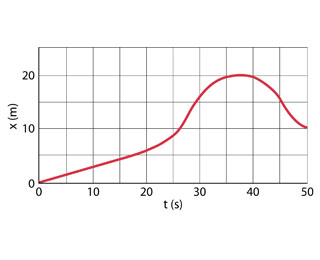# Problem: The position of a rabbit along a straight tunnel as a function of time is plotted in the figure.a.) What is its instantaneous velocity at t = 10.0s?b.) What is its instantaneous velocity at t = 30.0s?

###### FREE Expert Solution

Velocity:

$\overline{){\mathbf{v}}{\mathbf{=}}\frac{\mathbf{∆}\mathbf{x}}{\mathbf{∆}\mathbf{t}}}$

a)

Considering a tangent line at t = 10.0 s, we get the instantaneous velocity from the slope of the tangent line.

83% (251 ratings)###### Problem Details

The position of a rabbit along a straight tunnel as a function of time is plotted in the figure.a.) What is its instantaneous velocity at = 10.0s?

b.) What is its instantaneous velocity at t = 30.0s?